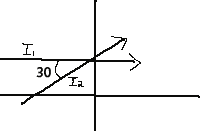# Flux and Current

timman_24

## Homework Statement

Two monoenergetic neutron beams I1=2*10^10 and I2=1*10^10 intersect at an angle of 30 degrees. Calculate both the flux and current in the region where they intersect.

## The Attempt at a Solution

I lined the first I1 up parallel to the x-axis and tilted I2 30 degrees below I1 to make the calculations easier.Flux:

I believe flux is the amount of neutrons passing between the left side to the right side across this arbitrary surface area. I took the surface area to span the y-z axis with the flux passing along the x-axis.

To calculate the flux, I simple did:

I1 + I2*cos(30) = 2.866*10^10 n/(cm^2-s)

Current:

I believe the current to be the vector representation of the net flow. For this I did the following:

J(Jx,Jy) = Jx + Jy
Jx= I1 + I2*cos(30)
Jy= I2*sin(30)

J(Jx,Jy) = 2.866*10^10 i +.5*10^10 j

I am not 100% positive if this is the correct way to do this. I get confused sometimes between current and flux.

Any help would be much appreciated!